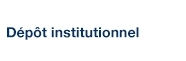Robustness to outliers in location–scale parameter model using log-regularly varying distributions

Desgagné, Alain (2015). « Robustness to outliers in location–scale parameter model using log-regularly varying distributions ». The Annals of Statistics, 43(4), pp. 1568-1595.

Fichier(s) associé(s) à ce document :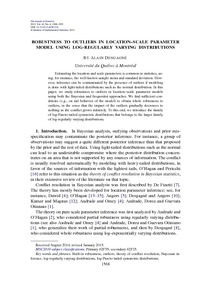Prévisualisation
PDF
Télécharger (287kB)

Résumé

Estimating the location and scale parameters is common in statistics, using, for instance, the well-known sample mean and standard deviation. However, inference can be contaminated by the presence of outliers if modeling is done with light-tailed distributions such as the normal distribution. In this paper, we study robustness to outliers in location–scale parameter models using both the Bayesian and frequentist approaches. We find sufficient conditions (e.g., on tail behavior of the model) to obtain whole robustness to outliers, in the sense that the impact of the outliers gradually decreases to nothing as the conflict grows infinitely. To this end, we introduce the family of log-Pareto-tailed symmetric distributions that belongs to the larger family of log-regularly varying distributions.

Type: Article de revue scientifique Built-in robustness, outliers, theory of conflict resolution, Bayesian inference, log-regularly varying distributions, log-Pareto-tailed symmetric distributions. Faculté des sciences > Département de mathématiques Alain Desgagné 18 avr. 2016 17:18 27 avr. 2016 18:24 http://archipel.uqam.ca/id/eprint/8175

Statistiques

Voir les statistiques sur cinq ans...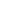RECHERCHER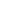PARCOURIR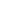LIBRE ACCÈS